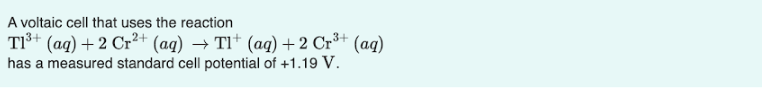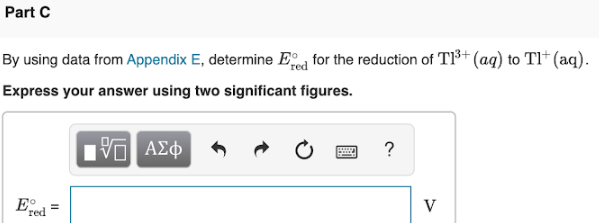# A voltaic cell that uses the reaction Tl^3+(aq) + 2Cr^2+(aq) → Tl^+ (aq) + 2 Cr^3+(aq) has measured standard cell potential of + 1.19 V. By using data from Appendix E, determine E°red for the reduction of Tl^3+ (aq) to Tl^+(aq). Express your answer using two significant figures.